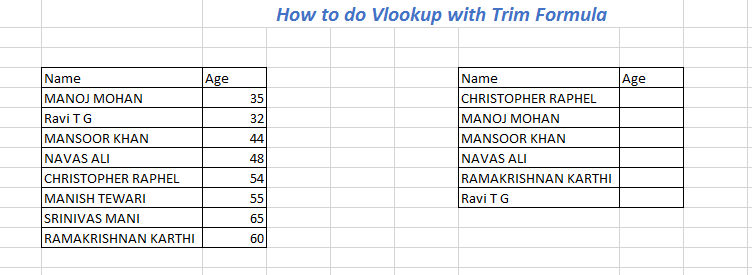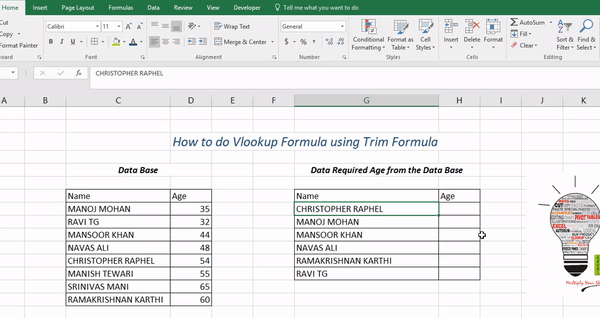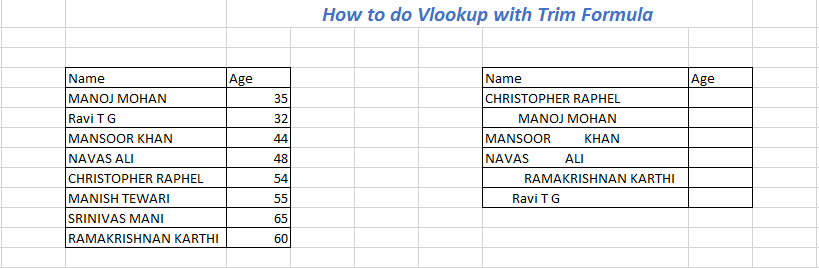# Blog#### How to do Trim with Vlookup Formula in ExcelWe know V lookup Formula is one of the most useful formula in Microsoft Excel but in some scenario we will face difficulty in getting results even our lookup value will be same , due to non visible spaces between the characters . In this scenario we can use trim function to solve this problem.

In this scenario the lookup value without extra spaces and here we can able see the result of the Vlookup Formula.In this Data we need to get the Age from the Data base where we can see the names of the employees are same , by applying the Formula :

=Vlookup Formula(Lookup Value (Names of Emloyees),Table Array(Data base of Employees with Age),Column index( Column number of Age from the Name),Exact Match(False/0).Vlookup Function with Trim Function

In this scenario the lookup value with extra spaces and here we will write trim function before the lookup value and now we will get the correct result.Formula:

=Vlookup Formula(Trim(Lookup Value (Names of Emloyees)),Table Array(Data base of Employees with Age),Column index( Column number of Age from the Name),Exact Match(False/0).